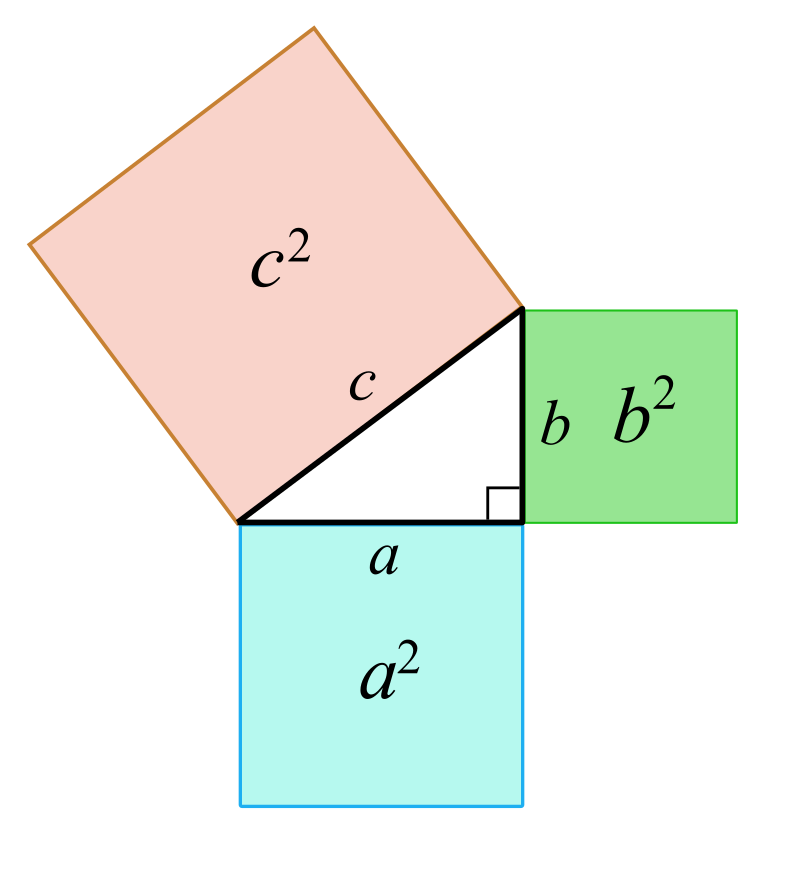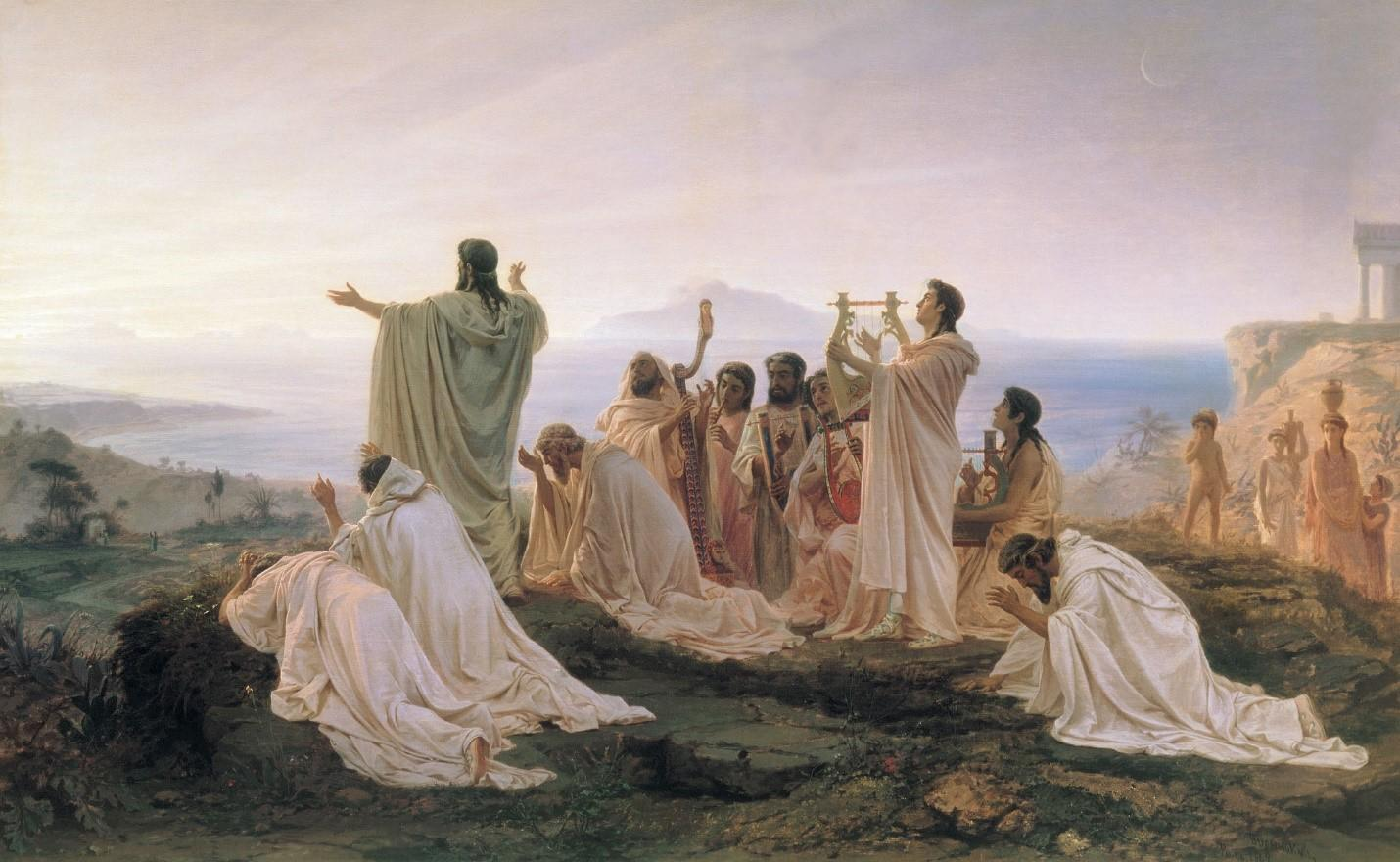Every year sees new reforms in the sector of education, reforms which strive to fit the changing values and interests of the government and students alike. It is no surprise that, with each new revision, there are some concepts that manage to stay a part of the maths curriculum. One such example is the concepts discovered and expanded by the great, mathematician Pythagoras. While he is most known for the theorem he gave his name to, the Pythagoras theorem, there still remains quite a bit of Pythagoras in our countless other maths exercises. Cosines, trigonometry, reciprocals, square roots, fractions, geometry, arithmetic, tangent, symmetry – discover the influence of Pythagoras in the history of maths! If you're interested in other famous Greek philosophers and mathematicians, check out Archimedes of Syracuse!

## The Early Life of Pythagoras

Born on the Greek island of Samos at the end of the 6th century BCE, Pythagoras is known as one of the greatest mathematicians from antiquity. However, the elements of his history have not been completely proven as no document from his era has survived. While some biographies that include Pythagoras’ life have been found, all were written well after Pythagoras died. Legend has it that some months before his birth, an oracle predicted the birth of a gifted being to Pythagoras’ father. This is how Pythagoras’ name was chosen, signifying “announced by Pythian.” Just as the oracle predicted, Pythagoras proved to be a very gifted child. History tells that at just eighteen years of age, Pythagoras not only participated in the Olympic games of combat, but he won all of the competitions. Athletic and intellectual, the young Pythagoras was interested as a scholar in Greek philosophy, history and the sciences. Some historians have also noted that he was the student of another celebrated mathematicians: Thales.Pythagoras has been the source of inspiration for many To continue his studies, the Greek philosopher and mathematician left on a series of journeys would eventually lead him to Egypt. There, he strove to learn from the teachings of the Egyptian priests until 525 BCE, when the Persians invaded the country and took him as a prisoner to Babylon. There, he utilized his time to study the knowledge of the Babylonian scribes. Once he was allowed to return to the island of Samos, Pythagoras started to teach in an amphitheater, but without much success. In fact, it was his attempts at spreading his knowledge that led to his banishment from the city. He decided to flee to Greater Greece in order to fulfill his goal of creating a school, giving lectures and continuing the tradition of Greek philosophers. Pythagoras made it a priority, throughout all of his life, to apply mathematics to philosophical thought. A number of disciples followed him in his experiences and research with the same passion – to the point where some have argued if Pythagoras started a sect and not a school. Pythagoras died around 500 BCE, but once again, the circumstances of his death are not well known.

## The History of the Pythagorean Theorem

Pythagoras has been the bane of many middle and high-schoolers' existence, with many struggling understand Pythagoras’ most seminal concept, the Pythagorean theorem. It must come as surprise, then, to learn that it was not actually discovered by Pythagoras. Engraved tablets of clay, dating back to 1800 BCE, illustrate that the length of triangles and their properties were already well known to the Babylonians, way before the arrival of Pythagoras. However, the reason Pythagoras has gained the credit for this theorem is because he was the first to define it in the way we still earn it today:

“For right triangles with the sides a, b and c, the length of the hypotenuse can be calculated by a2 + b2 = c2.”

Theorizing this law led Pythagoras to be forever remembered in the annals of history. To thank the gods for having helped him in his discovery, certain accounts hold Pythagoras to have sacrificed a hundred oxen. However, it was not enough to simply form the law into existence, Pythagoras had to prove his theory. The school founded by Pythagoras was in charge of this task, performing many experiments and calculations towards prove this theorem. The work done by these academics inspired countless other scientists, including even the infamous Leonardo de Vinci and Sir Isaac Newton.The Pythagorean theorem has led to many discoveries, such as the Pythagorean triple Without numbers, it is possible to also show the theorem like this:

“The sum of the squares of the lengths of a right triangle are equal to the square of its hypotenuse.”

This formula permits for the calculation of the length of one side of a right triangle, and simultaneously to prove that the triangle is a right triangle. In fact, if the square of the longest side is equal to the sum of the squares of the two other sides, the triangle is a right triangle. The theorem of Pythagoras, still taught today, led to the advancement of a particular type of geometry, known as “Euclidean geometry.” Get an online maths tutor now.

## Other Important Discoveries made by Pythagoras

Pythagoras was a veritable genius in many respects, and not just mathematics. According to him, mathematicians should strive to learn other subjects like music, philosophy, astronomy, mysticism. During the course of his life, he and his disciples adopted this mentality and managed to bring to light many mathematical discoveries. Most notably, Pythagoras discovered the link between the length of a vibrating string and the pitch of the note played on that string. His investigations in to music didn’t stop there, he also played with glasses containing different levels of water and found that each level of water represented a distinct musical note. These works were the basis of research of many other scientists and mathematicians, including Rene Descartes. His advancements in mathematics continued to permeate into many different disciplines and took on many different forms. Here are some of his most diverse discoveries. Pythagorean Spiral The Pythagorean spiral is a tool that allows for the geometric visualization of the square roots of consecutive whole numbers. To construct them, it is necessary to start by drawing a right triangle where the sides of the angle should be equal to 1. From this, basing the rest on Pythagoras’ theorem, the next step is to build a new triangle based on the hypotenuse of the first. One of the sides of the right angle of the new triangle has to be on the hypotenuse of the first. The other side of the angle should have the value of 1. The spiral forms by continuing the construction of the next right triangles in the same configuration as the ones proceeding it. Thanks to this tool, and the concept of right angles, we know the square roots of the consecutive whole numbers that are equal to the lengths of the hypotenuses of the right triangles. The Pythagorean Table of Opposites This table is largely utilized to teach multiplication tables to kids and adults. The Pythagorean table is simple: it deals with a table containing two columns. In the first column, on the left, values are written. These values are multiplied, added or divided, with the values to the right. The response is put on the intersection of the two values on the table. This table, utilized for multiplication, can also be used when there is a large amount of information that needs to be accounted for visually. Why not use this in your mathematics exercises to prepare for some upcoming exams? A maths tutorial might be helpful.

## The School of Pythagoreans in the Context of Mathematics

Pythagoras’ life goal was to teach math and to always have a group of people around him to help him in his scientific inquiries. This is why he found in Cortona, in the south of Italy, a school known as the School of Pythagoras. Likened to a sect, the members of this school discussed not only science but also aimed to be astute philosophers, politicians, and even involved in religion. In general, all of Pythagoras’ life centered around mathematics.The Pythagorean school was considered a sect by some For this school, numbers were the centre of everything. Pythagoras and his disciples continued to interest themselves in the divisibility of numbers and led to the famous proclamation: “numbers rule the universe.” The school lasted for 150 years and is totalled to have had about 218 members, members who were known as Pythagoreans. Because much of the work was executed as a team, it is impossible to know the exact identities of the real inventors of various formulas and principles we attribute to the Pythagorean school. They placed an emphasis on studying perfect numbers, associated numbers and sacred numbers. These latter were particularly appreciated by the Pythagoreans. For them, sacred numbers were made up of the sum of many exceptional numbers. The number 10, for example, could represent the sum of 4 prime numbers, the sum of a pair of squared prime numbers and one perfect number, or even the sum of the first three prime numbers. Pythagoras’ disciples and Pythagoras himself were sometimes considered as magicians by people in their epoque. This belief was mostly due to the fact that the Pythagoreans had discovered a way to trace a regular pentagon, a figure whose five sides are equal. To do this, the small team utilized five equilateral triangles that formed one pyramid with five faces. Once made, the scientists traced the base of the pyramid to obtain the pentagon. The pentagon that was traced by the figure was used as their symbol. The school of Pythagoreans was broken up after a dispute between two political factions within the group itself. Pythagoras and his school played a vital role in the evolution of mathematics. Whole numbers, circumferences, quadrilaterals, perpendicular, relative numbers, addition, parallelogram, decimals, sine, conjecture, scale products, fragment, diamond – all aspects of the math we learn today passed through the hand of the great mathematician.

Need a Maths teacher?5.00/5 - 1 vote(s)Loading...